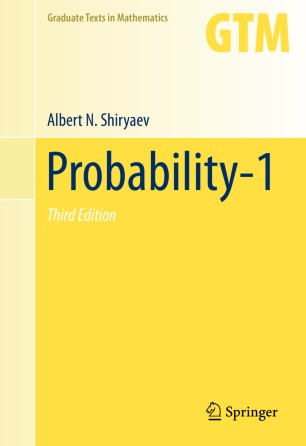# Probability-1

## Benefits

• Successfully synthesizes the most important classical ideas and results with many of the major achievements of modern probability theory

• Author provides clear and comprehensive introduction to probability theoryTextbook

Part of the Graduate Texts in Mathematics book series (GTM, volume 95)

1. Front Matter
Pages i-xvii
2. Albert N. Shiryaev
Pages 1-158
3. Albert N. Shiryaev
Pages 159-371
4. Albert N. Shiryaev
Pages 373-460
5. Back Matter
Pages 461-486

### Introduction

This book contains a systematic treatment of probability from the ground up, starting with intuitive ideas and gradually developing more sophisticated subjects, such as random walks, martingales, Markov chains, the measure-theoretic foundations of probability theory, weak convergence of probability measures, and the central limit theorem. Many examples are discussed in detail, and there are a large number of exercises. The book is accessible to advanced undergraduates and can be used as a text for independent study.

To accommodate the greatly expanded material in the third edition of Probability, the book is now divided into two volumes. This first volume contains updated references and substantial revisions of the first three chapters of the second edition. In particular, new material has been added on generating functions, the inclusion-exclusion principle, theorems on monotonic classes (relying on a detailed treatment of “π-λ” systems), and the fundamental theorems of mathematical statistics.

### Keywords

Ergodic theory Markov chain Martingale Probability theory Random variable Random walk Stochastic process Stochastic processes

#### Authors and affiliations

1. 1.Department of Probability Theory and Mathematical StatisticsSteklov Mathematical Institute and Lomonosov Moscow State UniversityMoscowRussia

Albert N. Shiryaev is Chief Scientific Researcher and Professor of Probability Theory and Mathematical Statistics at the Steklov Mathematical Institute of the Russian Academy of Sciences and Head of the Department of Probability Theory in the Mechanics and Mathematics Faculty at Lomonosov Moscow State University. He is the author of several books, including Problems in Probability [translated by Andrew Lyasov], Optimal Stopping Rules [translated by A.B. Aries], and Statistics of Random Processes [with Robert S. Liptser]. He was the recipient of the A.N. Kolmogorov Prize of the Russian Academy of Sciences in 1994 and the A.A. Markov Prize in 1974.

Dmitry M. Chibisov is Leading Scientific Researcher and Professor of Probability Theory and Mathematical Statistics at the Steklov Mathematical Institute of the Russian Academy of Sciences. He is the Editor-in-Chief of the journal Mathematical Methods of Statistics.

### Bibliographic information

• Book Title Probability-1
• Book Subtitle Volume 1
• Authors Albert N. Shiryaev
• Series Title Graduate Texts in Mathematics
• Series Abbreviated Title Graduate Texts Mathematics
• DOI https://doi.org/10.1007/978-0-387-72206-1
• Publisher Name Springer, New York, NY
• eBook Packages Mathematics and Statistics Mathematics and Statistics (R0)
• Hardcover ISBN 978-0-387-72205-4
• Softcover ISBN 978-1-4939-7905-9
• eBook ISBN 978-0-387-72206-1
• Series ISSN 0072-5285
• Series E-ISSN 2197-5612
• Edition Number 3
• Number of Pages XVII, 486
• Number of Illustrations 39 b/w illustrations, 0 illustrations in colour
• Additional Information Originally published in one volume. Translated from the 4th Russian edition (2007); 3rd Russian Edition 2004, 2nd Russian Edition 1989, 1st Russian Edition 1980. Previous English translations: 2nd Edition 1996, 1st Edition 1984.
• Topics
• Buy this book on publisher's site
Industry Sectors
Pharma
Biotechnology
IT & Software
Telecommunications
Electronics
Energy, Utilities & Environment
Aerospace
Oil, Gas & Geosciences
Engineering

## Reviews

“This book is axiomatic and abstract, and presents a comprehensive but pure-math approach to probability theory. … There are good exercises throughout the book … . I think this is a good book if you like this kind of very abstract approach.” (Allen Stenger, MAA Reviews, August, 2017)

“This book provides a general introduction to probability theory, and covers several advanced topics. … Numerous examples and problems help the reader to understand the topics. The book is recommended to master and PhD students in mathematics.” (László Viharos, Acta Scientiarum Mathematicarum, Vol. 83 (1-2), 2017)

It is clear that this book contains important and interesting results obtained through a long time period, beginning with the classical Bernoulli's law of large numbers, and ending with very recent results concerning convergence of martingales and absolute continuity of probability measures. Let us note especially that the great number of ideas, notions and statements in the book are well-motivated, explained in detail and illustrated by suitably chosen examples and a large number of exercises. Thus, the present book is a synthesis of all significant classical ideas and results, and many of the major achievements of modern probability theory. In the whole it is a welcome addition to mathematical literature and can become an indispensable textbook for courses in stochastics.

- J. Stoyanov, Zentralblatt